# Python Datetime Vs. NumPy Datetime: 8 Differences You Should Know

## How Python datetime and NumPy datetime modules handle the datetime values.### Introduction

Python datetime module helps in handling datetime values. These values are of the type DateTime class format. This DateTime format is nothing but a data type class that has additional accessors and attributes such as adding time differences, changing timezones, etc.

NumPy datetime objects are used by almost every individual using Pandas. Although Pandas has its class called Timestamps, which under the hood, uses the best of the two: NumPy vectorized interface and Python datetime ease of use. There are differences in how native Python datetime and NumPy datetime64 handle data. In this article, I will discuss some of these distinctions.

#### 1. Data Representation

Native Python and NumPy both follow different formats to store date-time attributes. The Python datetime format stores data as a group of integers for every bit of information. It means that year, month, day, minute, hour, seconds, and so on have their integer representation.

Interestingly, the NumPy follows an offset representation using the Unix epoch. The most common epoch is 1st January 1970. It calculates the datetime based on the number of seconds past the epoch. These values are stored in a signed int64 array.

To have a better understanding, you can visit Epoch Converter, and try different epochs to get different datetime. For example, here I have passed the epoch as the number of seconds in a day to get the next date post the baseline.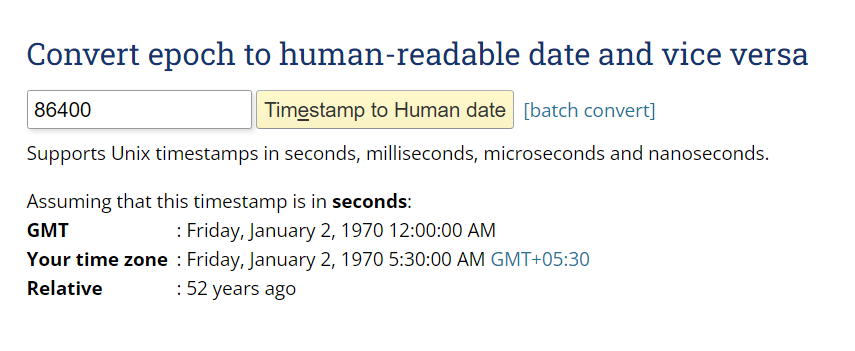Passing epoch value as the number of seconds in a day to get the next day post the base epoch. Image from the Epoch Converter website

Similarly, you can try the vice versa of converting the human-readable date to an epoch value.

#### 2. Time Delta & Resolution Granularity

Time deltas allow us to move dates forward or backward by the defined value. Python datetime supports time deltas up to limited units starting the week to just microseconds. NumPy supports 13 units from Year to Attoseconds for time deltas and therefore offers a deeper shift of dates.

Another difference between Python and NumPy datetime is the resolution. In Python, the data resolution may not be the same as the unit but NumPy data resolution is the same as the unit.

Let's understand this a bit deeper. For example, if we check the resolution for a Python datetime object with only date, it defaults the resolution to ‘days’ and if we check resolution for Python datetime object with time, suppose hour, it defaults the resolution to ‘microseconds’ instead of ‘hour’. It makes the Python datetime objects resolution inconsistent with the units provided. See the code example below: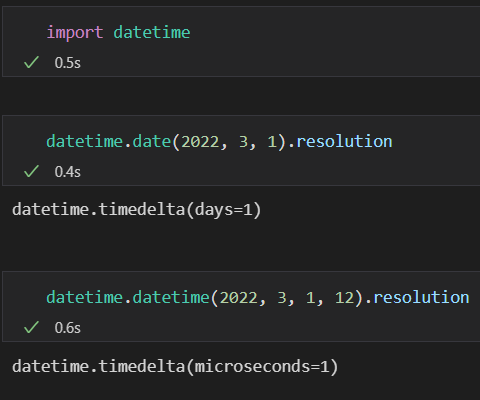Checking Python datetime objects resolution

In the case of NumPy datetime, the resolution is the same as the units provided. The resolution for NumPy objects can be seen using the ‘dtype’ attribute. This also shows that NumPy embeds the units as resolutions.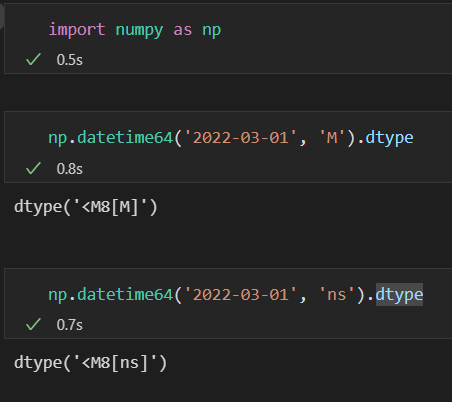Checking NumPy datetime object resolution

#### 3. Range

The range of native Python datetime has been set, hardcoded, between 1 to 9999 Years. It is done so because the Python integer can support a very large number, which is not achievable as of now. In the case of NumPy datetime, the range is flexible and a bit dynamic. The range is dependent on the int64 range along with the unit's range. Smaller units generally have a smaller range and so does the datetime range.

Have a look at Python datetime min-max dates: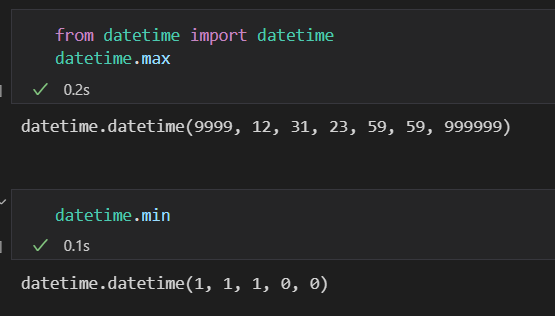Python Min-Max datetime objects

In the case of NumPy, the range decreases with the usage of smaller units. In the example below, you can see the max NumPy datetime with ‘day’ as a unit. When a lower unit, microseconds is chosen, the max date is decreased as well as time elements are introduced.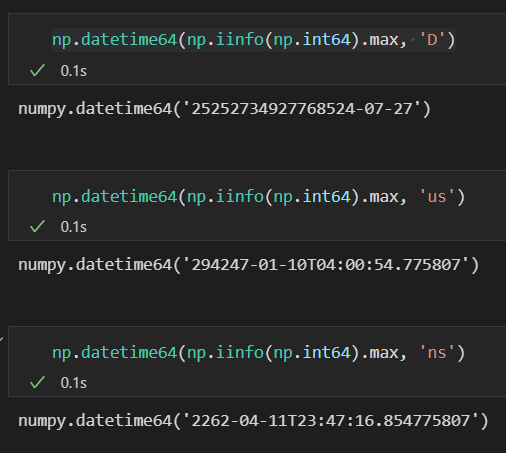NumPy datetime Min-Max ranges dependent on units chosen

#### 4. Converting in Other Units

We usually convert/streamline our datetime units to a standard unit so that the values are compatible for further calculations. In Python datetime, we create a new object for converting into other units. On the other hand, in NumPy datetime, we can simply use the “astype” method for converting between any unit. Here, the ease of usage aligns towards NumPy datetime.

See an example below where we converted the NumPy datetime default units into months and microseconds.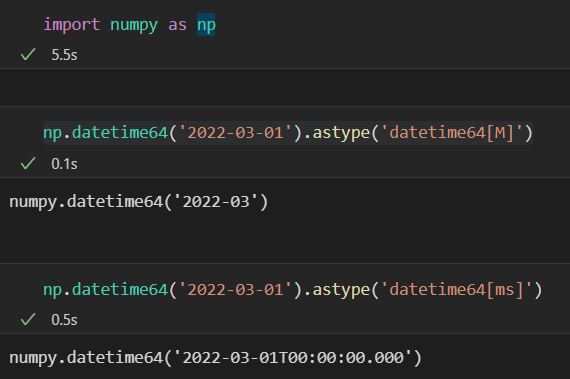Using NumPy astype method to convert default datetime unit into different units

#### 5. Shifting Using Arithmetic operators

This difference sets apart the NumPy datetime from Python datetime. NumPy supports operator overloading of addition and subtraction for integers. This means along with time delta support for datetime objects, you can add/sub numbers directly from datetime objects considering the unit defined. On the other hand, Python datetimes only support time delta add/sub. See the examples below: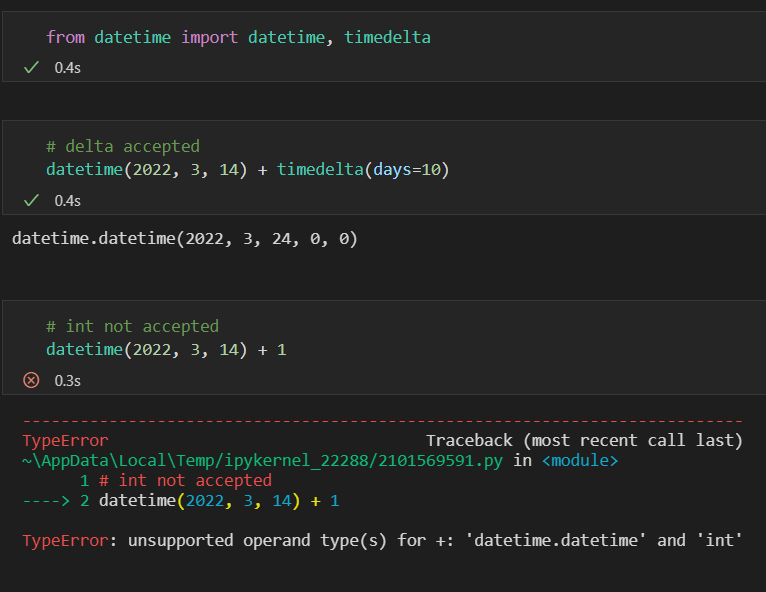Python datetime only support add/sub for time deltas and not the ‘int’ types

In the case of NumPy, operator overloading is accepted. Also, as NumPy supports vectorization, you can simply add/sub integers from a NumPy array of datetime objects, making it super easy.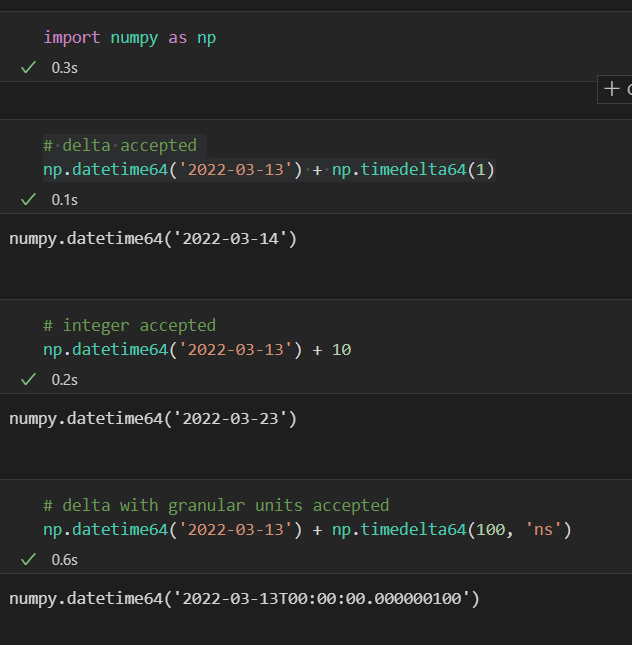NumPy supports time delta, as well as integers, add/sub

#### 6. Missing Values

We all are aware of the fact that NumPy and Pandas are known to handle missing values with ease. We have the “NaN” which represents a “not a number” placeholder for any type of missing data. This placeholder is special as it propagates with calculations and does not throw exceptions.

Similarly, in the case of datetime values, we have a special “NaT’, not a time placeholder that can be present in the NumPy datetime arrays. It smartly converts “None” or empty strings into the “NaT’ placeholder.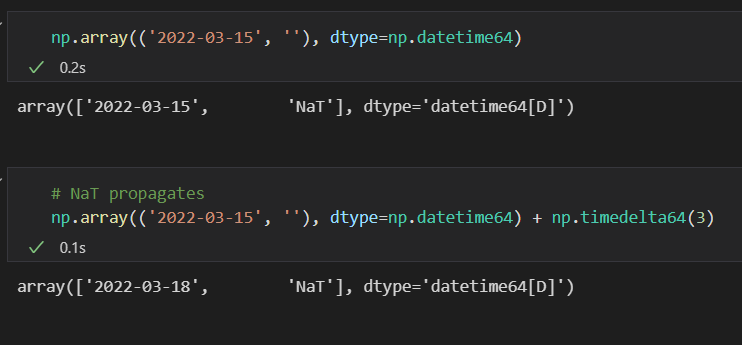‘NaT’ placeholder in NumPy for missing values

On the other hand, Python datetime serves missing values as “None” as the placeholder. The problem with this placeholder is that it does not propagate to further calculations and throws exceptions.

#### 7. Performance Comparisons

The NumPy is known to be a better Python implementation in terms of performance and speed. In this case, too, NumPy datetime is way faster than the Python datetime objects. In terms of applying operations on a list/array of datetime objects, NumPy leads as its core implementation is C-arrays which provide the much-needed vectorization. Great comparison with concrete numbers can be found here.

#### 8. Interconversion

Till now, we have seen that the NumPy datetimes have a border range as compared to the Python datetimes. NumPy has support for the input of smaller range datetime objects and Python datetime is no exception to this.

Therefore, if we want to convert a Python datetime object into a NumPy datetime object, it can be easily done by passing this Python object directly to the NumPy object and adding the “astype” method to apply the appropriate datetime data type unit. See one example below: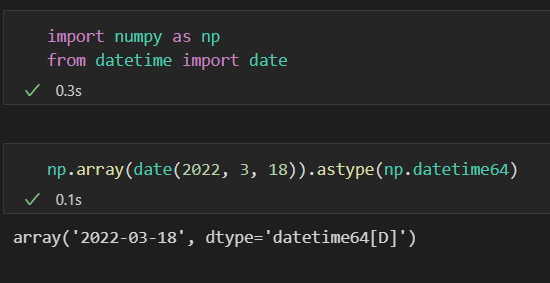Converting Python datetime into NumPy datetime

Now, when we move from a broader range of units to a narrow range, some of the unit's information would be distorted or transformed into offsets. NumPy handles it very gracefully and does not require any extra effort. It provides the astype and tolist method to convert a NumPy datetime to Python datetime. If you want to use the astype method, then you can simply pass the object as a parameter in astype and the resultant would be the Python datetime. Take a look at the below examples: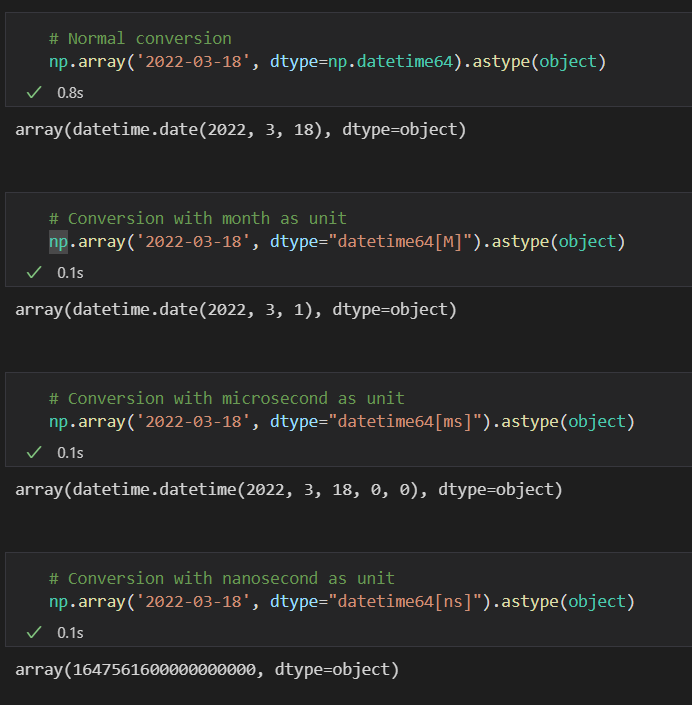Converting NumPy datetime into Python datetime

Note: Any unit conversion going below microseconds returns the offset representation.

### EndNotes

This article presents the differences between Python datetime and NumPy datetime objects. I think it is important to understand how these two modules handle the datetime values as it helps in implementing robust code. The combination of the two interfaces, pandas Timestamps is the perfect example of using the best of both worlds: NumPy vectorization and Python datetime ease of use. You can explore more about these differences and do let me know if I missed any differences!

If you want to read/explore every article of mine, then head over to my master article list which gets updated every time I publish a new article on any platform!

For any doubts, queries, or potential opportunities_, you can reach out to me via my LinkedIn

Previous Article:

Reference:

This article has been highly inspired and referred from this PyData Talk: